• ## Python列表推导式

万次阅读 多人点赞 2019-05-18 00:51:24
Python列表推导式 列表推导式是Python构建列表（list）的一种快捷方式,可以使用简洁的代码就创建出一个列表. 一、range()函数 python的range()函数可用来创建一个整数列表，一般用在 for 循环中. range()语法:...
Python列表推导式
列表推导式是Python构建列表（list）的一种快捷方式,可以使用简洁的代码就创建出一个列表.
一、range()函数
python的range()函数可用来创建一个整数列表，一般用在 for 循环中.
range()语法:range(start, stop[, step])
start: 计数从start开始,默认是从0开始(闭区间),如:range(5)等价于range(0,5).
stop: 计数到stop结束,但不包括stop(开区间).如:range(0,5)是[0, 1, 2, 3, 4],不包含5.
step:步长,相邻两个值的差值,默认为1.如:range(0,5)相当于range(0, 5, 1).
为什么要在列表推导式前讲range(),因为列表推导式是通过一个可迭代对象来生成列表的,range()可以说是列表推导式中最常用的可迭代对象了.对列表推导式来说,range()是其中的精髓之一.没有range(),列表推导式的可读性和简洁性将会大打折扣.
二、列表推导式
列表推导式(list comprehension)是指循环创建列表.
for循环有非常广的应用场景,也可以用来创建一个列表,而列表推导式就相当于for循环创建列表的简化版.
# for循环
list_a = list()
for a in range(5):
list_a.append(a)
print(list_a)
# 列表推导式
list_b = [b for b in range(5)]
print(list_b)
上面分别是用for循环和列表推导式创建列表的代码,list_a和list_b的结果是一样的,都是[0, 1, 2, 3, 4].
下面来看复杂点的列表推导式:
# in后面跟其他可迭代对象,如字符串
list_c = [7 * c for c in "python"]
print(list_c)

# 带if条件语句的列表推导式
list_d = [d for d in range(6) if d % 2 != 0]
print(list_d)

# 多个for循环
list_e = [(e, f * f) for e in range(3) for f in range(5, 15, 5)]
print(list_e)

# 嵌套列表推导式,多个并列条件
list_g = [[x for x in range(g - 3, g)] for g in range(22) if g % 3 == 0 and g != 0]
print(list_g)
运行结果:
['ppppppp', 'yyyyyyy', 'ttttttt', 'hhhhhhh', 'ooooooo', 'nnnnnnn']
[1, 3, 5]
[(0, 25), (0, 100), (1, 25), (1, 100), (2, 25), (2, 100)]
[[0, 1, 2], [3, 4, 5], [6, 7, 8], [9, 10, 11], [12, 13, 14], [15, 16, 17], [18, 19, 20]]
代码解释:
1.列表推导式会遍历后面的可迭代对象,然后按照for前的表达式进行运算,生成最终的列表.
2.如果有if条件语句,for遍历后紧跟着进行条件判断.
3.如果有多个for循环,则最终的数据数量为多个for循环的笛卡尔积.
4.可以进行嵌套的列表推导,与嵌套for循环的原理相同.
三、代码可读性
对于不熟悉列表推导式的人来说，可以使用for循环来实现相同的功能,但是需要好几行代码,而列表推导式只需要一行代码.
很多人会说代码简洁了但可读性降低了,其实不然,当我们对列表推导式熟悉(自己写几次就熟悉了)，代码的功能一眼就能轻松地看出来,但是for循环代码基本不可能一眼看完.尤其当创建列表的for循环嵌套在业务逻辑的其他for循环里时,恰恰是使用列表推导式的可读性最高.
同时,在Python3中列表推导式有自己的局部作用域,就像函数似的.表达式内部的变量和赋值只在局部起作用，表达式的上下文里的同名变量还可以被正常引用，局部变量并不会影响到它们.
也就是说,列表推导不会有变量泄漏的问题,在列表推导中的赋值操作不可能会影响到列表推导上下文中的同名变量.
当然,也不是所有场景都推荐使用列表推导式.比如说:如果列表推导的代码超过了两行,就要考虑改成用for循环了.超过了两行的列表推导式就真的没有可读性了.通常的原则是，只用列表推导来创建新的列表，并且尽量保持简短.
四、字典推导式
自Python2.7以来,列表推导概念就移植到了字典上,从而有了字典推导(后面还会看到集合推导).
如果列表推导的概念已经为你所熟知,接受字典推导并不难.直接看代码吧:
# 因为key是唯一的,所以最后value都是1
dict_a = {key: value for key in 'python' for value in range(2)}
print(dict_a)

# 可以根据键来构造值
dict_b = {key: key * key for key in range(6)}
print(dict_b)

# 遍历一个有键值关系的可迭代对象
list_phone = [('HUAWEI', '华为'), ('MI', '小米'), ('OPPO', 'OPPO'), ('VIVO', 'VIVO')]
dict_c = {key: value for key, value in list_phone}
print(dict_c)
运行结果:
{'p': 1, 'y': 1, 't': 1, 'h': 1, 'o': 1, 'n': 1}
{0: 0, 1: 1, 2: 4, 3: 9, 4: 16, 5: 25}
{'HUAWEI': '华为', 'MI': '小米', 'OPPO': 'OPPO', 'VIVO': 'VIVO'}
五、集合推导式
Python2.7不仅带来了字典推导式,还带来了集合推导式.
同样的,我们直接看代码,这样可以直观的看到集合推导式.
# 遍历一个可迭代对象生成集合
set_a = {value for value in '有人云淡风轻,有人负重前行'}
print(set_a)

运行结果:
{'负', '有', '人', '轻', '前', '云', '重', ',', '淡', '风', '行'}
集合是无序且不重复的,所以会自动去掉重复的元素,并且每次运行显示的顺序不一样.
从上面的代码中可以总结:
集合推导式就是将列表推导式的[]换成{},字典推导式就是推导出两个值并构建成键值对的样子.
另外,不管是字典推导式还是集合推导式,后面都可以像列表推导式一样接if条件语句,嵌套循环等,具体可以根据您自己的需求来使用.
关注工重号可以领资料和学习交流。展开全文• 目录   一.列表合并--append() 二.列表合并--extend() ...2.列表对象的append()可以将另一个列表追加到当前列表中,作为当前列表的一个元素。 二.列表合并--extend() 与append()不同，e...
目录

一.列表合并--append()
二.列表合并--extend()
三.列表合并--“+”
四.列表去重
五.列表排序

一.列表合并--append()
1.列表对象的append()可以将元素追加到列表中。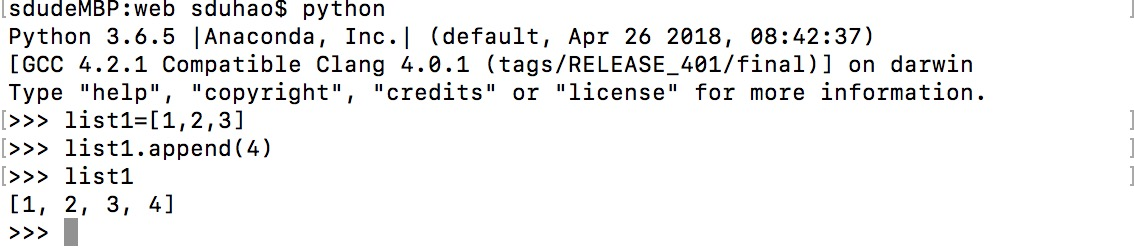2.列表对象的append()可以将另一个列表追加到当前列表中,作为当前列表的一个元素。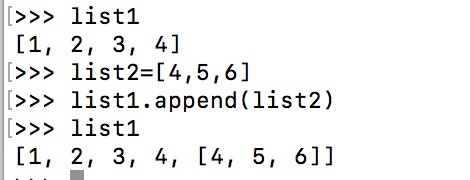二.列表合并--extend()
与append()不同，extend()可以将一个或多个元素追加到当前列表中。当追加多个元素时，参数为一个列表，与append()
不同的是，追加的列表不是整体作为一个元素追加到当前列表中，而是将其中的每个元素追加到当前列表中，相当于列表合并。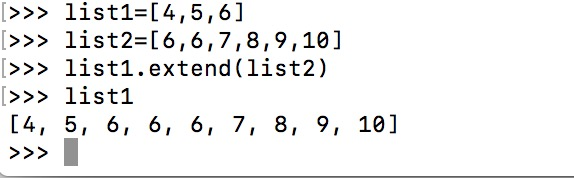三.列表合并--“+”
效果与extend()相同，也可用于列表的合并。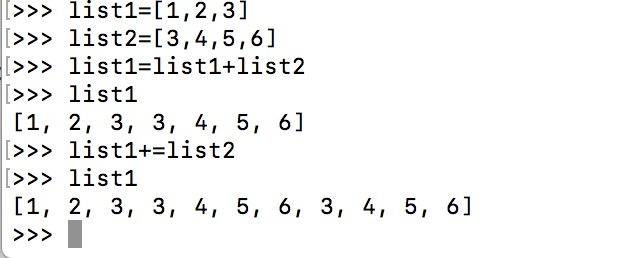四.列表去重
有时候合并完列表，我们需要对新列表的元素进行去重，此时可以使用set()。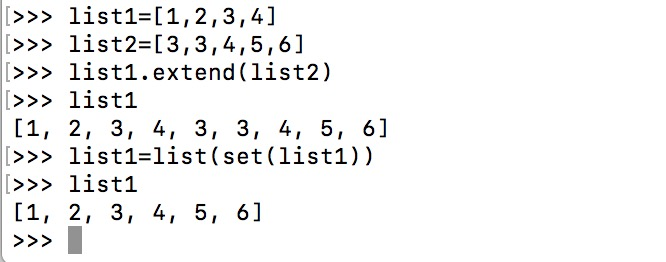五.列表排序
有时候合并完列表，我们需要对新列表的元素进行排序，此时可以使用列表对象的sort()或者sorted()。二者的不同是sort()是列表对象固有的方法，sorted()不仅针对列表，可以对所有序列进行排序。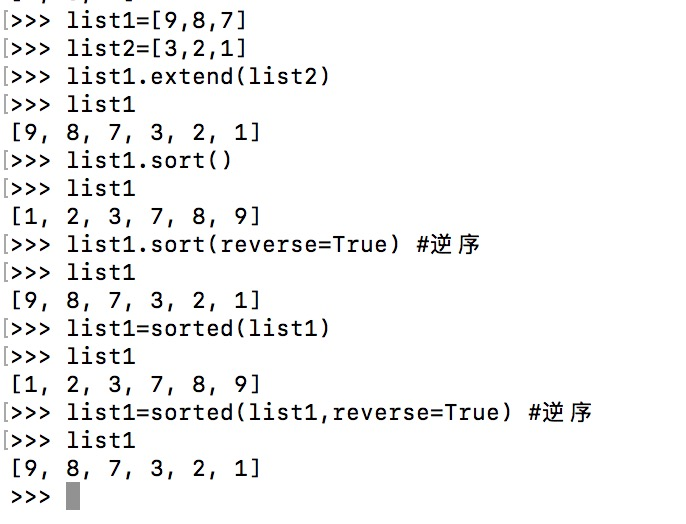展开全文• Python列表排序 list.sort方法和内置函数sorted 很多时候我们获取到一个列表后,这个列表并不满足我们的需求,我们需要的是一个有特殊顺序的列表. 这时候就可以使用list.sort方法和内置函数sorted,本文就是介绍list....
Python列表排序 list.sort方法和内置函数sorted
很多时候我们获取到一个列表后,这个列表并不满足我们的需求,我们需要的是一个有特殊顺序的列表.
这时候就可以使用list.sort方法和内置函数sorted,本文就是介绍list.sort方法和sorted内置函数的使用方法和区别.
一、list.sort方法
list.sort方法会就地排序列表，也就是说不会把原列表复制一份。这也是这个方法的返回值是None的原因，提醒您本方法不会新建一个列表。
在这种情况下返回None其实是Python的一个惯例：如果一个函数或者方法对对象进行的是就地改动，那它就应该返回 None，好让调用者知道传入的参数发生了变动，而且并未产生新的对象。
来看一下代码：
# coding=utf-8
list_a = [1, 2, 8, 3, 7, 9, 5, 7]
# sort()方法没有返回值
list_b = list_a.sort()
print("list_a: ", list_a)
print('list_b: ', list_b)
运行结果：
list_a:  [1, 2, 3, 5, 7, 7, 8, 9]
list_b:  None
用返回None来表示就地改动这个惯例有个弊端，那就是调用者无法将其串联起来。而返回一个新对象的方法则正好相反,它们可以链式调用，从而形成连贯接口。
二、sorted内置函数
与 list.sort 相反,内置函数sorted会新建一个列表作为返回值。
这个方法可以接受任何形式的可迭代对象作为参数，甚至包括不可变序列或生成器，而不管sorted接受的是怎样的参数，它最后都会返回一个列表。
代码示例:
list_c = [1, 2, 8, 3, 7, 9, 5, 7]
# sorted内置函数会返回一个排序后的新列表
list_d = sorted(list_c)
print("list_c: ", list_c)
print('list_d: ', list_d)
运行结果：
list_c:  [1, 2, 8, 3, 7, 9, 5, 7]
list_d:  [1, 2, 3, 5, 7, 7, 8, 9]
可以看到，使用内置函数sorted时，返回了一个新的列表，而原列表没有发生改变。
这有两种好处：
1.如果我们即需要使用原列表，也需要使用排序后的列表，或者说我们要将一个非列表的可迭代对象排序成列表，sorted都可以做到
2.有返回值时，我们可以进行链式调用
# 可以对非列表的可迭代对象排序生成列表
str_e = 'python'
list_e = sorted(str_e)
print(list_e)

# 链式调用
str_f = '-'.join(sorted(str_e)).upper().split('-')
print(str_f)

运行结果：
['h', 'n', 'o', 'p', 't', 'y']
['H', 'N', 'O', 'P', 'T', 'Y']
三、关键字参数key和reverse
不管是 list.sort 方法还是 sorted 函数，都有两个可选的关键字参数:
key： 接收一个只有一个参数的函数，这个函数会被用在序列里的每一个元素上，所产生的结果将是排序算法依赖的对比关键字。
比如说，在对一些字符串排序时，可以用 key=str.lower 来实现忽略大小写的排序，或者是用 key=len 进行基于字符串长度的排序。key的默认值是恒等函数，也就是默认用元素自己的值来排序。
reverse:
如果被设定为 True，被排序的序列里的元素会以降序输出(也就是说把最大值当作最小值来排序),reverse的默认值是 False.
phone = ('HUAWEI', 'OPPO', 'MI', 'MEIZU', 'VIVO')
# 按长度进行排序
phone_list = sorted(phone, key=len)
print(phone_list)

phone_list_re = sorted(phone, key=len, reverse=True)
print(phone_list_re)
运行结果：
['MI', 'OPPO', 'VIVO', 'MEIZU', 'HUAWEI']
['HUAWEI', 'MEIZU', 'OPPO', 'VIVO', 'MI']
上面的代码中，第一次排序新建了一个按照长度排序的字符串列表。第二次排序是将按长度排序由升序变成了降序。
细心的您应该可以发现，第二次的结果并不是第一次排序的结果的完全翻转。
OPPO和VIVO的长度都是4，reverse=True后，它们的相对位置跟第一次排序是一样的。这是什么原因呢？
sorted和list.sort背后的排序算法都是Timsort，它是一种自适应算法，会根据原始数据的顺序特点交替使用插入排序和归并排 序，以达到最佳效率。
Python的排序算法Timsort是稳定的（知道这一点就可以了），意思是就算两个元素比不出大小，在每次排序的结果里它们的相对位置是固定的。
因为用到的排序算法是稳定的，也就是说在长度一样时，OPPO和VIVO的相对位置不会改变。 关于list.sort()方法和sorted内置函数的使用，现在已经掌握了~展开全文内置函数sorted
• python列表查找值Hi Folks! In this article, we will have a look at the various ways to find the average of a list in a Python List. 嗨伙计！ 在本文中，我们将介绍在Python List中查找列表平均值的各种方法 ...

python列表查找值

Hi Folks! In this article, we will have a look at the various ways to find the average of a list in a Python List.
嗨伙计！ 在本文中，我们将介绍在Python List中查找列表平均值的各种方法 。
In general, an average is a value that represents a whole set of data items or elements.
通常，平均值是代表整个数据项或元素集的值。

Formula: Average = summation of numbers/total count.
公式：平均值=数字总和/总计数。

在Python中查找列表平均值的技巧 (Techniques to find the average of a list in Python)
Either of the following techniques can be used to calculate the average/mean of a list in Python:
以下任何一种技术均可用于计算Python中列表的平均值/均值：
Python mean() function Python mean（）函数 In-built sum() method 内置sum（）方法 Python lambda and reduce() method Python Lambda和reduce（）方法 Python operator.add() method Python operator.add（）方法

1. Python mean（）函数 (1. Python mean() function)
Python 3 has statistics module which contains an in-built function to calculate the mean or average of numbers. The statistics.mean() function is used to calculate the mean/average of input values or data set.
Python 3具有statistics module ，该statistics module包含一个内置函数来计算数字的均值或平均值。 statistics.mean() function用于计算输入值或数据集的平均值/平均值 。
The mean() function accepts the list, tuple or data-set containing numeric values as a parameter and returns the average of the data-items.
mean（）函数接受包含数值的列表，元组或数据集作为参数，并返回数据项的平均值。

Syntax:
句法：

mean(data-set/input-values)

Example:
例：

from statistics import mean

inp_lst = [12, 45, 78, 36, 45, 237.11, -1, 88]
list_avg = mean(inp_lst)

print("Average value of the list:\n")
print(list_avg)
print("Average value of the list with precision upto 3 decimal value:\n")
print(round(list_avg,3))


In the above snippet of code, we have used statistics.round() method to round off the output average up to a particular decimal value.
在上面的代码片段中，我们使用了statistics.round()方法将输出平均值四舍五入到特定的十进制值 。
Syntax:
句法：

statistics.round(value, precision value)

Output:
输出：

Average value of the list:

67.51375
Average value of the list with precision upto 3 decimal value:

67.514


2.使用Python sum（）函数 (2. Using Python sum() function)
Python statistics.sum()function can also be used to find the average of data values in Python list.
Python statistics.sum()函数还可用于在Python列表中查找数据值的平均值。
The statistics.len() function is used to calculate the length of the list i.e. the count of data items present in the list.
statistics.len()函数用于计算列表的长度，即列表中存在的数据项的数量。
Syntax:
句法：

len(input-list)

Further, statistics.sum() function is used to calculate the sum of all the data items in the list.
此外， statistics.sum()函数用于计算列表中所有数据项的总和。
Syntax:
句法：

sum(input-list)

Note: average = (sum)/(count).
注意： average =（sum）/（count） 。
Example:
例：

from statistics import mean

inp_lst = [12, 45, 78, 36, 45, 237.11, -1, 88]

sum_lst = sum(inp_lst)

lst_avg = sum_lst/len(inp_lst)
print("Average value of the list:\n")
print(lst_avg)
print("Average value of the list with precision upto 3 decimal value:\n")
print(round(lst_avg,3))

Output:
输出：


Average value of the list:

67.51375
Average value of the list with precision upto 3 decimal value:

67.514



3.使用Python reduce（）和lambda方法 (3. Using Python reduce() and lambda method)
We can use Python reduce() function along with the lambda() function.
我们可以将Python reduce（）函数与lambda（）函数一起使用。
Python reduce() function: The reduce() function is basically used to apply a particular(input) function to the set of elements passed to the function.
Python reduce（）函数 ： reduce() function基本上用于将特定（输入）函数应用于传递给该函数的元素集。
Syntax:
句法：

reduce(function,input-list/sequence)

Initially, the reduce() function applies the passed function to the first two consecutive elements and returns the result. 最初，reduce（）函数将传递的函数应用于前两个连续的元素，并返回结果。 Further, we apply the same function to the result obtained in the previous step and the element succeeding the second element. 此外，我们将相同的函数应用于上一步中获得的结果以及第二个元素之后的元素。 This process continues until it reaches the end of the list. 此过程一直持续到列表末尾。 Finally, the result is returned to the terminal/screen as output. 最后，结果作为输出返回到终端/屏幕。
Python lambda() function: The lambda() function is used to build and form Anonymous functions i.e. function without a name or signature.
Python lambda（）函数 ： lambda() function用于构建和形成匿名函数，即没有名称或签名的函数。
Syntax:
句法：

lambda arguments:function

Example:
例：

from functools import reduce

inp_lst = [12, 45, 78, 36, 45, 237.11, -1, 88]

lst_len= len(inp_lst)

lst_avg = reduce(lambda x, y: x + y, inp_lst) /lst_len
print("Average value of the list:\n")
print(lst_avg)
print("Average value of the list with precision upto 3 decimal value:\n")
print(round(lst_avg,3))


Output:
输出：

Average value of the list:

67.51375
Average value of the list with precision upto 3 decimal value:

67.514



4. Python operator.add（）函数查找列表的平均值 (4. Python operator.add() function to find the average of a list)
The Python operator module contains various functions to perform basic calculations and operations efficiently.
Python运算符模块包含各种功能，可以有效地执行基本计算和操作。
The operator.add() function can be used to calculate the summation of all the data values present in the list with the help of Python reduce() function.
借助Python reduce（）函数，可以使用operator.add()函数来计算列表中存在的所有数据值的总和。
Syntax:
句法：


Note: average = (sum)/(length or count of elements)
注意：平均值=（总和）/（元素的长度或数量）
Example:
例：

from functools import reduce
import operator
inp_lst = [12, 45, 78, 36, 45, 237.11, -1, 88]

lst_len = len(inp_lst)

print("Average value of the list:\n")
print(lst_avg)
print("Average value of the list with precision upto 3 decimal value:\n")
print(round(lst_avg,3))

Output:
输出：

Average value of the list:

67.51375
Average value of the list with precision upto 3 decimal value:

67.514


5. NumPy average（）方法来计算Python中列表的平均值 (5. NumPy average() method to calculate the average of a list in Python)
Python’s NumPy module has an in-built function to calculate the average/mean of the data items present in the data set or list.
Python的NumPy模块具有一个内置函数，用于计算数据集或列表中存在的数据项的平均值/均值。
The numpy.average() method is used to calculate the average of the input list.
numpy.average()方法用于计算输入列表的平均值。
Example:
例：

import numpy

inp_lst = [12, 45, 78, 36, 45, 237.11, -1, 88]

lst_avg = numpy.average(inp_lst)
print("Average value of the list:\n")
print(lst_avg)
print("Average value of the list with precision upto 3 decimal value:\n")
print(round(lst_avg,3))


Output:
输出 ：

Average value of the list:

67.51375
Average value of the list with precision upto 3 decimal value:

67.514

结论 (Conclusion)
Thus, in this article, we have unveiled and understood various techniques to find the average of a Python List.
因此，在本文中，我们揭示并理解了各种技术来查找Python列表的平均值。

参考资料 ()
展开全文• Python列表查重、获取列表中的重复元素、列表去重 #!/usr/bin/env python #!-*-coding:utf-8 -*- def run(lit): # 判断lit中是否有重复的元素 if [lit.count(x) for x in lit if lit.count(x) > 1]: lit1 = ...
• ## python列表相加

千次阅读 2020-04-23 19:09:14
1.若两列表长度相等，想得到两个列表每个元素相加结果： [a[i]+b[i] for i in range(len(a))] 2.两个列表直接使用+相加：效果类似于extend()函数 a=[1,2,3] b=[4,5,6] print(a+b) ...
• Python列表操作大全（非常全！！！）对于python列表的理解可以和C语言里面的数组进行比较性的记忆与对照，它们比较相似，对于python里面列表的定义可以直接用方括号里加所包含对象的方法，并且python的列表是比较...
• python实现列表对应元素求和的两种方法。 方法一：采用zip（）方法： 1 2 3 4 5 6 7 8 9 10 11 12 # -*...
• python列表的增删改1、list增加元素1.1 append（）1.2 extend（）1.3 insert（）1.4 切片 1、list增加元素 python中列表增加元素有四种方式： append()：在列表末尾添加一个元素 extend()：在列表末尾添加至少一个...
• sum([1, 2, 3, 4]) # 结果为10 sum([1, 2, 3], 4) # 结果也为10
• ## python列表元素的积

千次阅读 2019-10-17 15:32:39
python列表元素的积 描述 一个由n(n>1)个数字组成的列表 ls，输出一个列表lt，其中lt中第i个元素等于ls中除ls[i]之外所有元素的乘积。 输入输出均为列表形式。试例： 输入 输出 [1,2,3,4] [24,12,8,6] ...
• ## python列表的逆序遍历

千次阅读 多人点赞 2020-04-18 13:36:00
拿到这道题，题目的意思已经很清楚了，列表里含有2的元素都需要删除，然后输出删除后的新列表。我首先想到的思路就是使用for循环遍历字符串，利用字符串操作符x in s（如果x是s的子串，返回True，否则返回False），...字符串
• python 获取唯一值In this article, we will be understanding 3 ways to get unique values from a Python list. While dealing with a huge amount of raw data, we often come across situations wherein we need...java 机器学习 数据分析
• ## Python列表长度

万次阅读 2018-03-28 17:27:33
确定列表长度 # 定义一个汽车品牌数组 cars = ['bmw', 'audi', 'benz'] # 输出列表长度 print('列表长度是:') print(len(cars)) 输出 列表长度是: 3
• ## Python列表反转显示方法

千次阅读 多人点赞 2020-01-15 14:53:11
第一种，使用reversed 函数，reversed返回的结果是一个反转的迭代器，我们需要对其进行 list 转换 listNode = [1,2,3,4,5...第二种，使用sorted函数，sorted是排序函数，它是对一个列表进行排序后生成一个新的list列...编程语言
• python 列表list中元素添加的四种方式（append，extend，insert，“+”号） 1.append() 追加单个元素到List的尾部，只接受一个参数，参数可以是任何数据类型 。 2.extend()将一个列表中每个元素分别添加到另一个...
• ## Python列表函数&方法

千次阅读 多人点赞 2018-10-16 20:18:06
Python包含以下函数: 函数 都是python内置的函数 len(list) 列表元素个数 max(list) 返回列表元素最大值 min(list) 返回列表元素最小值 list(seq) 将元组转换为列表 Python包含以下方法: 方法 list.append(obj) ...列表的方法
• ## python列表取值

千次阅读 2019-12-04 20:09:06
# python 列表取值 list = ['张三','李四','王五','赵六','钱七','孙八'] print(list) zhaoliu = list print(zhaoliu) zhaoliu =list[-3] print(zhaoliu) # 范围取值:列表变量 = 原来表变量[起始索引：结束索引]...练习
• python列表中随机选择Python random module provides an inbuilt method choice() has an ability to select a random item from the list and other sequences. Using the choice() method, either a single random...人工智能 java 机器学习
• 有时候可能要修改python列表，通过遍历整个列表，修改值。但是这里有一个问题。 直接看代码吧。 num_list =range(5) for item in num_list: item = item*2 print num_list 这样循环取里面的元素，修改里面的...队列 java 数据结构
• python 列表 分割 list.split( ) list.split() 就是以字符串或列表里某个字符把字符串或列表进行分割成新的字符串或列表。 list.split(“，”) 这就是以“，”来分割，如果原来字符串或列表里没有“，” 则无法...字符串 plist cstring
• 例如输入列表: [8, 2, 4] 期望输出: [1, 2, 0] 一句话可以搞定： rank = [index for index, value in sorted(list(enumerate(input_list)), key=lambda x:x)] 实例： input_list = [8, 2, 4] rank = [index for ...排序 索引
• Python中是有查找功能的，四种方式：in、not in、count、index； 下面以 List = ["L","rong","wu","forever"] 为例 （1）in字符 ：判断值是否在列表中 （2）not in...
• 一句话，对Python列表使用乘法，对于不可变对象（如数字、字符串）而言是复制值，但对可变对象（如列表、字典）而言则是复制引用，因此对于包含可变对象的列表切莫使用列表乘法，可使用列表生成式代替。简单例子见下...
• ## python列表添加数据

万次阅读 2018-11-19 16:18:28
python列表里面添加数据的方法以及各种方法时所用时间 循环 arr=[] for item in range(1000): arr.append(item) 加法 arr=[] for item in range(1000): arr=arr+[item] range的方法 list(range(1000)) ...添加数据 运行效率
• 另外附加两个python的for循环的操作 一个是for循环可以遍历字符串还可以遍历元祖 x = ['runoob', '123', '456'] for each in x: print(each) 输出: runoob 123 456 x = 'runoob' for i in range(len(x)) :...for循环
• 如何将两个列表组合生成字典？ #convert list to dict list1 = [1, 2, 3] #list1 print(list1) list2 = [“one”, “two”, “three”] #list2 print(list2) obj_zip = zip(list1,list2) #zip object print...
• python 列表乘一个数字 在python中, 如果用一个列表list1乘一个数字n 会得到一个新的列表list2, 这个列表的元素是list1的元素重复n次, 例如 list1 =  list2 = list1 * 5 # list2 = [0, 0, 0, 0, 0] 但如果...
• 你是否还在为列表中的nan而苦恼，跟随我的脚步，两步告别万千烦恼丝。 import numpy as np list_test = ['a', 'b', 'c', np.nan] print(list_test) while np.nan in list_test: list_test.remove(np.nan) print...
• ## python列表截取操作

千次阅读 2019-05-09 09:42:36...

# python列表python 订阅